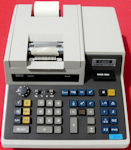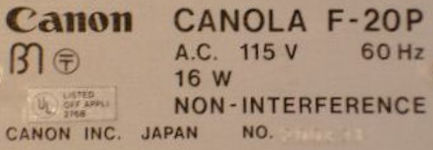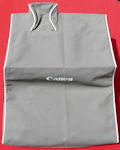Vintage Electronic Calculators:CALCUSEUM Home page Overview of Brands Colleagues CANON: F20P(Page 1/1)AKA: Canola F-20P, AKA (Label): CANOLA F-20P, Product number (P/N): F20P (F-20P), Keywords/Tags: F20P (F-20P) | Canola Date of intro: 1976, Origin: Japan (List), Dimensions: 330x254x102mm, Power: AC, Number of keys: 42, #Key-Black: 6, #Key-Blue: 15, #Key-Grey: 17, #Key-Yellow: 4, Decimal switch: [0-1-2-3-4-5-6-F], Round switch: [5/4-(ArrowDown)], Miscellaneous switch: [x.y], Classification: / Desktop, Featuring: Scientific functions, Programmable, Memories: 1, Certification: 276B(UL) (List All UL's), Collector value: 8/10, Info: Thanks to HESS Duane for following analyzing (03-oct-2017)...Its quite interesting how it represents numbers. Its a 16 digit, print only machine, but you only see 12 digits at a time.There is no scientific notation.You can manually enter 16 digits total. 16 to the left of the decimal point.Only 15 to the right as the implied leading '0.' takes up one position even though keying the leading zero is unnecessary.16 digits when numbers are split left & right of the decimal point.Entering more than 15 digits to the right of the decimal produces overflow.Entering or calculating more than 16 digits to the left of the decimal produces overflow. Calculations with more than 15 digits to the right of the decimal are dropped after the 15th digit.How (or if) a decimal point is printed clues you to whether more digits are available. You then need to multiply or divide by multiples of 10 and manually subtract what you see in order to view remaining digits. At this point manual tracking of the decimal point is required.There are no punctuation marks, but will add them for clarity:- a result (or manual entry) of 1,234,567,890,123,456. prints as 123456789012-- as the decimal is missing, that is the clue there are more digits, so arbitrarily devide by 100,000.-- you will then see 12345678901.2-- subtracting 12345678901 produces 0.23456- a result of exactly zero prints as: 0.-- zero with a decimal point- a result (or manual entry of) of 0.000,000,000,000,001 will print as .000000000000-- decimal point with 12 zeros.-- multiply by 1,000,000 produces 0.000000001-- i.e. 0.000,000,001- a result of 0.123,456,789,012,345 will print as .123456789012-- subtracting .123456789012 produces .000000000000Addition and subtraction is adding machine style so the above example would be handled as:-- calculation/manual entry producing 0.123456789012345 prints as .123456789012-- press the '+' key-- key in .123456789012-- press the '-' (minus) key-- pressing the '=' key (labelled */=) produces .000000000000-- multiply by 1000000 (1,000,000)-- pressing the '=' key produces 0.000000345 (0.000,000,345)I would need to go through every function on the unit to document fully, but the built in functions produce either 10 or 16 digit results; depending on the function. For example log and antilog (base e) produce 10 digit results. Interestingly log(e) will accept more than 9 digits to the right of the decimal and will produce a more accurate 10 digit result. Likewise with antilog (e). The antilog(e) tests performed were of the nature 1.nnn,nnn,nnn,n & more decimal digits. Log(e) tests had varing number of digits to the left & right of the decimal. Cursory comparisons with modern 30 digit calculators implies 1 extra digit (maybe 2) is utilized for logarithmic input values. Whether an extra guard digit is also used in internal calculations was hard to determine.Due to the extensive testing needed with modern calculators for I didn't mess with it that much.The statistical functions which were checked produce 16 digit results.1/x and square root produce 16 digit results; but not always.SQRT(2) produces 16 digits.SQRT (2000) produces 16 digits.However SQRT (2100) produces 15 digits.As with many other functions a LOT of further testing is needed to decipher its behavior.a(x) - raising to powers produce 10 digit results.Undoubtedly logs (probably base e) are used to calculate powers. As, typical for the age, many calculators could not raise a negative number to a power. i.e. (-2)^3 produces overflow, not -8. Minor testing implies an extra guard digit is either used in internal calculations or extra iterative steps are used in its algorithms producing a guard digit to round the result to 10 digits. Major testing is needed for a full description and I have not done such.Depending on the x/x,y switch the SUM key performs the standard summations of unpaired (x) and paired (x,y) data. Other calculators often have Sigma+ for the nomenclature of this key.SigmaOUT produces the standard n, sum(x), sum(x^2), sum(y), sum(y^2), sum(x*y).I have no idea how to operate the distribution (bell curve thing) nor z-statistic functions. Output of single result stat functions remain in the keyboard input buffer for further manual or preprogrammed function usage. Same applies to multi-result stat functions with the last output being available. Naturally, in either case, keying in a new number overwrites the input buffer.The only way to clear the statistic registers is via CA (clear all).Unfortunately, CA produces only 'C' for output, as does C (clear), so there is no visual indication on the paper tape which was pressed.I do not feel confident about understanding the DEL key (delete statistical data point entries).Other calculators often have Sigma- for the nomenclature of this key.The DEL removes the last x or x,y values entered after summation (pressed the SUM key) but appears you must do so immediately before entering further values. If you enter incorrect values then continue summing further values I have no idea how you "go back" to correct that situation.-- for unpaired data press the DEL key immediately after pressing SUM for the x value. Output will be 'Delete X'. Pressing DEL after keying in the x value but before pressing SUM behaves as if you pressed the CE key (clear entry) but produces the additional output 'Delete', you may then re-enter the value.-- for paired data---- pressing DEL after keying in a x value and have pressed SUM produces 'Delete X'. Pressing DEL after keying a x value but have not pressed SUM behaves the same as unpaired data description above.---- pressing DEL after keying in a y value and pressing SUM produces 'Delete X' 'Delete Y'. The entire pair is deleted. Pressing DEL after keying a y value but have not pressed SUM behaves as the unpaired description above and awaits another y value.There is only one manual memory register and behaves adding machine style.S being recall (RM) and T being recall then clear memory (RM + CM).There are 13 print positions for numbers and the decimal point.Positive numbers print in black and negative in red, as usual. Negative numbers have a left-most floating minus sign.When all 13 positions are used for digits and decimal point the minus sign is omitted and the value prints in red. ItemLabel

Protection (dust cover)Created: 04-jun-2014, Manual-update: 09-oct-2017, Batch-update: 19-jun-2021 # Pageloads since 2018: 35 3 Pic(s), If you have a (better) personal picture and/or missing info about any model not listed: Please do send a Mail